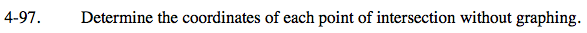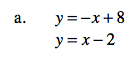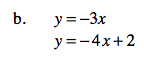### Home > CAAC > Chapter 4 > Lesson 4.2.4 > Problem4-97

4-97.Use the Equal Values Method.

Substitute your answer for x back into one of the original equations to find y.

(5, 3)

y = y
x + 8 = x − 2

x + 8 = x − 2
+2 +2

x + 10 = x
+x +x

10 = 2x

5 = x

y = −x + 8
y = −(5) + 8
y = 3Follow the steps outlined in part (a).

(2, −6)